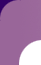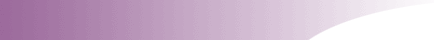ASL University | Checklist | Lessons | Signs | Products | Resources | LifeprintASL 101 Lesson 45 Quiz:Lesson 45 Quiz:  Instructions:  Choose the single most correct response: 1.   a.   b.   c.   d.   2.   a.   b.   c.   d.   3.   a.   b.   c.   d.   4.   a.   b.   c.   d.   5.   a.   b.   c.   d.   6.   a.   b.   c.   d.   7.   a.   b.   c.   d.   8.   a.   b.   c.   d.   9.   a.   b.   c.   d.   10.   a.   b.   c.   d. © Lifeprint Institute